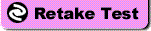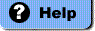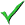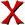# Chemistry Quiz

True/False

Indicate whether the sentence or statement is true ' + 'or false.

'; instructionText = '

Multiple Choice

Identify the letter of the choice that best ' + 'completes the statement or answers the question.

'; instructionIndex = 0; instructionIndex = 10; narrativeText = ''; narrativeIndex = 16; numberText = '1.'; questionText = '

The types of ' + 'particles that are located in the nucleus of an atom are protons and neutrons, and the types of ' + 'particles that are located in the energy levels surrounding the nucleus are electrons.

T

'; numberText = '2.'; questionText = '

The atomic ' + 'number of carbon is 6, so it must contain 12 electrons.

F

'; numberText = '3.'; questionText = '

Most elements ' + 'are stable if their outermost energy level contains an even number of electrons.

F

'; numberText = '4.'; questionText = '

Atoms in a ' + 'gas move more rapidly than atoms in a liquid or a solid do.

T

'; numberText = '5.'; questionText = '

The products ' + 'of an endergonic chemical reaction possess more energy than the reactants from which they are ' + 'produced.

T

'; numberText = '6.'; questionText = '

The amount ' + 'of energy needed to cause a chemical reaction to start is called activation energy.

T

'; numberText = '7.'; questionText = '

When an enzyme ' + 'binds with its substrate, the activation energy needed for the chemical reaction to occur is ' + 'raised.

F

'; numberText = '8.'; questionText = '

Enzymes speed up a chemical reaction by increasing the activation energy of the ' + 'reaction.

F

'; numberText = '9.'; questionText = '

Free hydrogen ' + 'ions can react with water molecules and form a positively charged ion, the hydronium ' + 'ion.

T

'; numberText = '10.'; questionText = '

Buffers can neutralize acids, but they do not affect bases.

F

'; numberText = '11.'; questionText = '

The smallest ' + 'particle of matter that can retain the chemical properties of carbon is

 a. a carbon molecule. c. a carbon atom. b. a carbon macromolecule. d. an element.

C

'; numberText = '12.'; questionText = '

The bond formed ' + 'when two atoms share a pair of electrons is called a

 a. hydrogen bond. c. covalent bond. b. nonpolar bond. d. water bond.

C

'; numberText = '13.'; questionText = '

Sharing of electrons in the outer energy levels of two atoms

 a. results in ion formation. b. occurs in covalent bonds. c. only occurs if both are atoms of the same ' + 'element. d. is found only ' + 'among carbon atoms.

B

'; numberText = '14.'; questionText = '

An atom that ' + 'has gained or lost electrons is called a(n)

 a. molecule. c. ion. b. nucleon. d. element.

C

'; numberText = '15.'; questionText = '

Which of the ' + 'following statements most accurately describes the difference between an ionic bond and a covalent ' + 'bond?

 a. Atoms held together by ionic bonds separate when placed in water while ' + 'atoms held together by covalent bonds do not separate in water. b. Ionic bonds hold together atoms of two different types, while covalent ' + 'bonds hold together atoms of the same type. c. Electrons are exchanged between atoms held together by an ionic bond, ' + 'but they are shared between atoms held together by a covalent bond. d. Ionic bonds form between atoms that carry opposite charges, while ' + 'covalent bonds form between uncharged atoms.

C

'; numberText = '16.'; questionText = '

A reaction ' + 'in which the products have less energy than the reactants is

 a. an endergonic reaction. c. a filamentous reaction. b. an exergonic reaction. d. impossible.

B

'; numberText = '17.'; questionText = '

Refer to the ' + 'graph above. Reaction “1” in the graph

 a. is an energy-storing reaction. b. requires a greater activation energy than Reaction ' + '“2.” c. may use the same ' + 'initial reactant condition needed to form Product “B.” d. All of the above

D

'; numberText = '18.'; questionText = '

Refer to the ' + 'graph above. Which of these statements is true regarding the graph?

 a. Reaction “2” occurs faster than Reaction “3” ' + 'because Reaction “2” requires more energy than Reaction ' + '“3.” b. The difference in ' + 'the graphs shown for Reaction “2” and Reaction “3” is due to a difference in the ' + 'activation energy needed for these reactions. c. Reactant “A” contains more energy at the beginning of the ' + 'reaction than Product “C” has after the reaction. d. All of the above

B

'; numberText = '19.'; questionText = '

Changing the course or pathway of a chemical reaction so that it requires less ' + 'activation energy

 a. is a violation of the laws of nature. b. requires higher temperatures than those found within ' + 'cells. c. occurs only when ' + 'reactants are quickly added to the reaction mixture. d. is accomplished by the action of catalysts on ' + 'reactants.

D

'; numberText = '20.'; questionText = '

Oxidation-reduction reactions are important in organisms because they

 a. allow the passage of energy from molecule to ' + 'molecule. b. prevent nuclear ' + 'reactions from occurring. c. allow the creation and destruction of energy. d. None of the above; oxidation-reduction reactions do not occur in living ' + 'organisms.

A

'; studentName = StripSpaces(f.student_name.value); FixMTF(f); index = 0; for (i=0; i 0) { text = StripSpaces(GetResponse(index, f.elements[i])); if (text.length == 0) ++numBlank; ++index; } } if (studentName == "") { alert("Student name cannot be blank."); return(false); } if (numBlank > 0) { if (numBlank == 1) msg = "1 question"; else msg = numBlank + " questions"; if (!confirm("You have not answered " + msg + " Are you sure you want to end the test?")) return(false); } index = 0; for (i=0; i 0) { text = GetResponse(index, f.elements[i]); if (ansMap[index] == "") { prefixText[index] = ""; ++numSubjective; } else { isCorrect = ScoreAnswer(index, text); if (isCorrect) ++numCorrect; ++numPossible; prefixText[index] = BuildPrefixText(index, text, isCorrect); } responseText[index] = TranslateHtmlString(text); ++index; } } if (showNotes) { for (i=0; i 0) scorePercent = Math.round(100.0 * numCorrect / numPossible); else scorePercent = 0; studentName = TranslateHtmlString(studentName); document.writeln("n

"); document.writeln("" + titleString + ""); document.writeln("

n"); document.writeln("

"); document.writeln("Name: " + studentName + "
"); document.write("Score: " + numCorrect + " / " + numPossible + " (" + scorePercent + "%)"); if (numSubjective > 0) { document.write("     [" + numSubjective + " subjective "); if (numSubjective == 1) document.write("question"); else document.write("questions"); document.write(" not graded]"); } document.writeln(" "); document.writeln("

" + titleString + "

"); document.writeln("

"); currentInstruction = 0; currentNarrative = 0; for (i=0; i

"); ++currentInstruction; } } if (currentNarrative

"); ++currentNarrative; } } document.writeln("

"); document.writeln("

"); document.writeln("

"); document.writeln("

"); } document.writeln("

"); document.writeln(instructionText[currentInstruction]); document.writeln("

"); document.writeln("

"); document.writeln(narrativeText[currentNarrative]); document.writeln("

"); document.writeln("

" + prefixText[i] + "

" + numberText[i] + "

" + questionText[i]); document.writeln("

"); document.writeln("

"); if (listMap.charAt(i) != "1") { document.writeln("

"); document.writeln("

"); } document.writeln("

"); document.writeln("

"); if (showNotes) { document.writeln("

"); document.writeln("

"); } if (showRef) { document.writeln("

"); document.writeln("

"); } document.writeln("

 RESPONSE: " + responseText[i] + " ANSWER: " + answerText[i] + " NOTES: " + notesText[i] + " REF: " + refText[i] + "

"); document.writeln("

"); document.writeln("
"); document.writeln("

"); document.writeln("

"); document.write("

"); document.writeln("

 "); document.writeln(""); document.write(""); document.writeln(""); document.writeln("

`"  		if (isCorrect)  			text += ""  		else  			text += ""    		if (listMap.charAt(answerIndex) == "1") {  			if (responseText.length == 0)  				responseText = " ";  			text += " " + responseText + " ";  		}    		text += "`

" return(text); } function StripSpaces(s) { var len; var i; len = s.length; for (i=len - 1; i >= 0 && s.charAt(i) == " "; --i) len = i; if (len == 0) s = ""; else if (len != s.length) s = s.substring(0, len); return(s); } function TranslateHtmlString(text) { var newText; var replaceString; var position; var length; var breakPos; var skipExtra; var i; newText = ""; position = 0; length = text.length; while (position ': replaceString = ">"; breakPos = i; break; case '"; breakPos = i; break; case 'n': replaceString = "
"; breakPos = i; break; case ' ': if ((i + 1 position) newText += text.substring(position, breakPos); newText += replaceString; position = breakPos + 1 + skipExtra; } } return(newText); } function FixMCNotes(notesText, studentText) { var displayText; var searchText; var upperNotes; var charCode; var startIndex; var endIndex; displayText = ""; if (studentText.length > 0 && notesText.length > 0) { upperNotes = notesText.toUpperCase(); studentText = studentText.toUpperCase(); charCode = studentText.charCodeAt(0); searchText = "/" + String.fromCharCode(charCode) + "/"; startIndex = upperNotes.indexOf(searchText); if (startIndex >= 0) { startIndex += searchText.length; searchText = "/" + String.fromCharCode(charCode + 1) + "/"; endIndex = upperNotes.indexOf(searchText, startIndex); if (endIndex = "0" && ch = 0) { len = s1.length; for (i=len - 1; i >= decimalPos; --i) { if (i == decimalPos || s1.charAt(i) == '0') len = i; else break; } if (len 0) { if (numToDelete == s1.length) --numToDelete; if (numToDelete > 0) s1 = s1.substring(numToDelete); } ///////////////////////////////////////////// tempString = ""; for (i=0; i = "0" && ch = 0) { len = s2.length; for (i=len - 1; i >= decimalPos; --i) { if (i == decimalPos || s2.charAt(i) == '0') len = i; else break; } if (len 0) { if (numToDelete == s2.length) --numToDelete; if (numToDelete > 0) s2 = s2.substring(numToDelete); } if (s1Sign == s2Sign && s1 == s2) return(true); } return(false); } function MultiCompare(responseText, answerText) { var startIndex; var endIndex; var partialText; responseText = responseText.toUpperCase(); answerText = answerText.toUpperCase(); startIndex = 0; do { endIndex = answerText.indexOf("r", startIndex); if (endIndex 0); return(false); } function FixMTF(f) { var text; var letter; var theList; var listIndex; var number; var i; for (i=0; i

 Name:  Chemistry QuizTrue/False
Indicate whether the sentence or statement is true or false.
1.
The types of particles that are located in the nucleus of an atom are protons and neutrons, and the types of particles that are located in the energy levels surrounding the nucleus are electrons.
2.
The atomic number of carbon is 6, so it must contain 12 electrons.
3.
Most elements are stable if their outermost energy level contains an even number of electrons.
4.
Atoms in a gas move more rapidly than atoms in a liquid or a solid do.
5.
The products of an endergonic chemical reaction possess more energy than the reactants from which they are produced.
6.
The amount of energy needed to cause a chemical reaction to start is called activation energy.
7.
When an enzyme binds with its substrate, the activation energy needed for the chemical reaction to occur is raised.
8.
Enzymes speed up a chemical reaction by increasing the activation energy of the reaction.
9.
Free hydrogen ions can react with water molecules and form a positively charged ion, the hydronium ion.
10.
Buffers can neutralize acids, but they do not affect bases.

Multiple Choice
Identify the letter of the choice that best completes the statement or answers the question.
11.
The smallest particle of matter that can retain the chemical properties of carbon is
 a. a carbon molecule. c. a carbon atom. b. a carbon macromolecule. d. an element.
12.
The bond formed when two atoms share a pair of electrons is called a
 a. hydrogen bond. c. covalent bond. b. nonpolar bond. d. water bond.
13.
Sharing of electrons in the outer energy levels of two atoms
 a. results in ion formation. b. occurs in covalent bonds. c. only occurs if both are atoms of the same element. d. is found only among carbon atoms.
14.
An atom that has gained or lost electrons is called a(n)
 a. molecule. c. ion. b. nucleon. d. element.
15.
Which of the following statements most accurately describes the difference between an ionic bond and a covalent bond?
 a. Atoms held together by ionic bonds separate when placed in water while atoms held together by covalent bonds do not separate in water. b. Ionic bonds hold together atoms of two different types, while covalent bonds hold together atoms of the same type. c. Electrons are exchanged between atoms held together by an ionic bond, but they are shared between atoms held together by a covalent bond. d. Ionic bonds form between atoms that carry opposite charges, while covalent bonds form between uncharged atoms.
16.
A reaction in which the products have less energy than the reactants is
 a. an endergonic reaction. c. a filamentous reaction. b. an exergonic reaction. d. impossible.17.
Refer to the graph above. Reaction “1” in the graph
 a. is an energy-storing reaction. b. requires a greater activation energy than Reaction “2.” c. may use the same initial reactant condition needed to form Product “B.” d. All of the above
18.
Refer to the graph above. Which of these statements is true regarding the graph?
 a. Reaction “2” occurs faster than Reaction “3” because Reaction “2” requires more energy than Reaction “3.” b. The difference in the graphs shown for Reaction “2” and Reaction “3” is due to a difference in the activation energy needed for these reactions. c. Reactant “A” contains more energy at the beginning of the reaction than Product “C” has after the reaction. d. All of the above
19.
Changing the course or pathway of a chemical reaction so that it requires less activation energy
 a. is a violation of the laws of nature. b. requires higher temperatures than those found within cells. c. occurs only when reactants are quickly added to the reaction mixture. d. is accomplished by the action of catalysts on reactants.
20.
Oxidation-reduction reactions are important in organisms because they
 a. allow the passage of energy from molecule to molecule. b. prevent nuclear reactions from occurring. c. allow the creation and destruction of energy. d. None of the above; oxidation-reduction reactions do not occur in living organisms.

Posted on